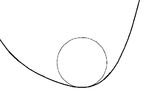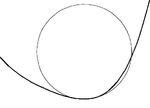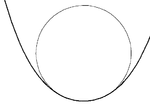Contact (mathematics)

﻿
Contact (mathematics)

In mathematics, contact of order k of functions is an equivalence relation, corresponding to having the same value at a point P and also the same derivatives there, up to order k. The equivalence classes are generally called jets. The point of osculation is also called the double cusp.

One speaks also of curves and geometric objects having k-th order contact at a point: this is also called osculation (i.e. kissing), generalising the property of being tangent. See for example osculating circle and osculating orbit.

Contact forms are particular differential forms of degree 1 on odd-dimensional manifolds; see contact geometry. Contact transformations are related changes of co-ordinates, of importance in classical mechanics. See also Legendre transformation.

Contact between manifolds is often studied in singularity theory, where the type of contact are classified, these include the A series (A0: crossing, A1: tangent, A2: osculating, ...) and the umbilic or D-series where there is a high degree of contact with the sphere.

Contact between curves

Two curves in the plane intersecting at a point p are said to have:

• 1-point contact if the curves have a simple crossing (not tangent).
• 2-point contact if the two curves are tangent.
• 3-point contact if the curvatures of the curves are equal. Such curves are said to be osculating.
• 4-point contact if the derivatives of the curvature are equal.
• 5-point contact if the second derivatives of the curvature are equal.

Contact between a curve and a circleA circle with 2-point contact (tangent)A circle with 3-point contact (osculating)A circle with 4-point contact at a vertex of a curve

For a smooth curve S in the plane then for each point, S(t) on the curve then there is always exactly one osculating circle which has radius 1/κ(t) where κ(t) is the curvature of the curve at t. If the curve has zero curvature (i.e. an inflection point on the curve) then the osculating circle will be a straight line. The set of the centers of all the osculating circles form the evolute of the curve.

If the derivative of curvature κ'(t) is zero, then the osculating circle will have 4-point contact and the curve is said to have a vertex. The evolute will have a cusp at the center of the circle. The sign of the second derivative of curvature determines whether the curve has a local minimum or maximum of curvature. All closed curves will have at least four vertices, two minima and two maxima (the four-vertex theorem).

In general a curve will not have 5-point with any circle. However, 5-point contact can occur generically[disambiguation needed] in a 1-parameter family of curves, where two vertices (one maximum and one minimum) come together and annihilate. At such points the second derivative of curvature will be zero.

Bi-tangents

It is also possible to consider circles which have two point contact with two points S(t1), S(t2) on the curve. Such circles are bi-tangent circles. The centers of all bi-tangent circles form the symmetry set. The medial axis is a sub set of the symmetry set. These sets have been used as a method of characterising the shapes of biological objects.

Wikimedia Foundation. 2010.

Look at other dictionaries:

• Contact — For information on contacting Wikipedia, see Wikipedia:Contact us. Contact may refer to: Contents 1 Social interaction 2 Mathematics and engineering 3 …   Wikipedia

• Contact geometry — Contact form redirects here. For a web email form, see Form (web)#Form to email scripts. The standard contact structure on R3. Each point in R3 has a plane associated to it by the contact structure, in this case as the kernel of the one form dz − …   Wikipedia

• Contact (novel) — Contact   …   Wikipedia

• Mathematics and Physical Sciences — ▪ 2003 Introduction Mathematics       Mathematics in 2002 was marked by two discoveries in number theory. The first may have practical implications; the second satisfied a 150 year old curiosity.       Computer scientist Manindra Agrawal of the… …   Universalium

• mathematics, philosophy of — Branch of philosophy concerned with the epistemology and ontology of mathematics. Early in the 20th century, three main schools of thought called logicism, formalism, and intuitionism arose to account for and resolve the crisis in the foundations …   Universalium

• mathematics — /math euh mat iks/, n. 1. (used with a sing. v.) the systematic treatment of magnitude, relationships between figures and forms, and relations between quantities expressed symbolically. 2. (used with a sing. or pl. v.) mathematical procedures,… …   Universalium

• mathematics, East Asian — Introduction       the discipline of mathematics as it developed in China and Japan.       When speaking of mathematics in East Asia, it is necessary to take into account China, Japan, Korea, and Vietnam as a whole. At a very early time in their… …   Universalium

• MATHEMATICS — Bible The Bible does not deal directly with proper mathematical subjects; however there are some parts that do relate indirectly to different mathematical topics. These are widely discussed by the various commentators on the Bible and Talmud: the …   Encyclopedia of Judaism

• mathematics, South Asian — Introduction       the discipline of mathematics as it developed in the Indian (India) subcontinent.       The mathematics of classical Indian civilization is an intriguing blend of the familiar and the strange. For the modern individual, Indian… …   Universalium

• Mathematics Subject Classification — The Mathematics Subject Classification (MSC) is an alphanumerical classification scheme collaboratively produced by staff of and based on the coverage of the two major mathematical reviewing databases, Mathematical Reviews and Zentralblatt MATH.… …   Wikipedia